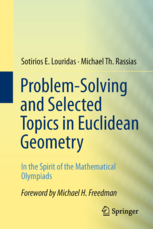Review of Problem-Solving and Selected Topics in Euclidean Geometry In the Spirit of the Mathematical Olympiads Sotirios E. Louridas, Michael Th. RassiasProblem-solving is an activity of solving problems; the skill of problem-solving is the ability to approach solving problems in a systematic manner. The skill - either innate or acquired - may fade unless exercised. Sotirios E. Louridas and Michael Th. Rassias, the authors of the book at hand, put together an excellent collection of problems to practice, and detailed solutions to follow the masters exposing that skill. If the title of the book is any indication, they set out to achieve more - probably, to instruct in problem-solving, or, at least, outline their problem-solving methodology. The structure of the book also points in this direction.

The short first chapter consists of two sections: "The Origins of Geometry" and "A Few Words About Euclid's Elements", each two-pages long; the former including the motivation and statement of Fermat's Last Theorem.

The short second chapter also consists of two parts. In the first part, the authors introduce logical connectives and truth tables; use these subsequently to define the relation between a theorem and its inverse, converse, and contrapositive statements and also introduce the concepts of the necessary and sufficient. In the second part, they describe four methods of proof: proof by analysis, proof by synthesis, proof by contradiction, and proof by induction. This is an interesting and unusual classification. Commonly, a proof may consists of two stages: analysis and synthesis. In the authors terminology, "proof by analysis" and "proof by synthesis", each refer to one complete process of proving. The latter starts with a known fact and proceeds by constructing a sequence of necessary conditions that culminate in the one that had to be proved. In proof by analysis, one starts with the to-be-proved proposition and goes backwards finding sufficient propositions at every step of the chain until arriving at the conditions of the problem.

I have two observations concerning second chapter. The first is a trifling matter: I have not found a problem in the book that is solved with the mathematical induction. Why is it mentioned? The second is more to the point. The process of proving, i.e., of actually putting down a proof, is likely the last stage in solving a problem. The delivery of necessary conditions in a proof by synthesis and sufficient ones in a proof by analysis needs to be guided by a deliberate, systematic search that picks up possible candidate propositions out of a well designed tool chest and verifies them against the present advance of the solution. The four-item classification is too broad to account for that. A well-stocked problem-solving tool chest contains many items: generalization, relaxation, pattern recognition, similarity, continuity, transformation, and more. The authors are of course well aware of that and this is why the third (30-page) chapter is devoted to geometric transformations and the next (20-page long) one lists many useful theorems, some with very detailed proofs.

Thus, chapter 3 presents plane geometric transformations, each applied to solve a sample problem or two, with a special emphasis on the inversion in circle. The chapter ends with an enlightening section that illustrates the development of an olympiad problem from a simpler one through a series of modifications.

The fourth chapter presents essential theorems of Euclidean Geometry. The list is reasonably complete. Regarding this chapter, I have two reservations. The first, again, is a trifle: some theorems are misnamed (i.e., Pappou (p. 75), instead of Pappus, common in the English speaking world), some are cited without usual attribution (i.e., Monge's (77-78), Miquel's theorems (p. 63), Regiomontanus' problem (p. 134), Broken Chord Theorem (69-71)); some statements are designated as corollaries (p. 72), although I was unable to identify the theorems whose corollaries they are. There are a few other peeves that I do not care to mention. One essential grievance was caused by an erroneous proof of my beloved Morley's theorem. As I recently saw a proof with a very similar starting point, I suspect that the proof in the book has been garbled in the editor, but that the authors are in possession of a correct version.

Chapter 5 is a problem collection, chapter 6 is a collection of solutions. The two chapters are split into three sections each: Geometric Problems with Basic Theory, Geometric Problems with More Advanced Theory, Geometric Inequalities. The collections are excellent, though not big enough (about 70 problems altogether) to be systematic. Some of the advanced problems are at the IMO level. The solutions are well thought of and their presentation is clear.

My reservations about some aspects of the book should not detract from the fact that an active reader would greatly benefit from reading the book; while working out the problems is bound to sharpen his or her problem solving skills. This is not an all-in-one manual of problem-solving, but it's a worthy addition to a library of a problem solver.

The book is referred to atProblem-Solving and Selected Topics in Euclidean Geometry, Springer (May 17, 2013). Softcover, 260 pp, \$49.99. ISBN 1461472725.